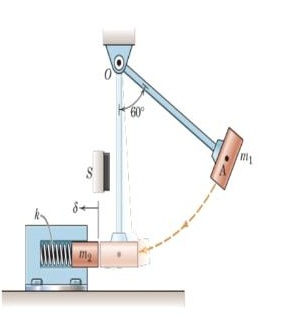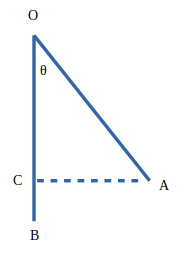# The pendulum is released from 60^0 position and then strikes the initially stationary cylinder...

## Question:

The pendulum is released from {eq}60^0 {/eq} position and then strikes the initially stationary cylinder of mass {eq}m_2 {/eq} When OA is vertical. Determine the maximum spring compression {eq}\delta {/eq} when {eq}m_1=3kg,m_2=2kg,OA=0.8m,k=6kN/m {/eq}

Assume the bar of the pendulum is light so that the mass {eq}m_1 {/eq} is effectively concentrated at point A The rubber cushion S stops the pendulum just after the collision is over. Neglect friction.## Conservation of Energy:

In an isolated system ( when neither mass or energy can get in or get out of the system ) total mass and energy are conserved. In modern physics, both mass and energy are interchangeable which was given by the famous equation of Einstein i.e. {eq}E=mc^2 {/eq}. But in classical mechanics, we can conserve mass and energy separately. Sometimes energy gets dissipated due to some nonconservative forces such as friction. The energy loss due to such forces doesn't get destroyed. It just gets lost in the form of heat and radiation.

## Step 1:

Let's analyze the situation first. The progress of transfer of energy will be from potential energy of mass 1 to the kinetic energy of mass 1 to the kinetic energy of mass 2 to compression of the spring. But, as there are no dissipative forces in the system, we can directly conserve the energy i.e. change in potential energy will be completely used in the compression of spring energy.

## Step 2:

We need to find the height to determine the potential energy. Which means we need to find OC (refer to the figure below).Using geometry, we can resolve OA into sine and cosine of the angle {eq}\begin{align*} \theta \end{align*} {/eq}.

Therefore,

{eq}\begin{align*} &CB = OB - OC\\ \Rightarrow &CB = OB - OA \cos\theta \\ \end{align*} {/eq}

It is given that OA= 0.8m and {eq}\begin{align*} \theta = 60^{\circ} \end{align*} {/eq}. Also OB = OA.

That's why,

{eq}\begin{align*} &CB = 0.8 - 0.8\cos 60\\ \Rightarrow & CB = 0.4 \end{align*} {/eq}

Let CB = h.

## Step 3:

We can now simply conserve the energy in the system.

{eq}\begin{align*} &m_1\times g\times h=\frac{1}{2}\times k\times \delta^2\\ \Rightarrow & 3\times 9.8 \times 0.4 = \frac {1}{2} \times 6000 \times \delta^2 \\ \Rightarrow & \delta^2 = 0.00392 \\ \Rightarrow & \delta = \sqrt{0.00392}\\ \Rightarrow & \delta = 0.0626099\\ \end{align*} {/eq}

The maximum spring compression will be 0.0626099 m.